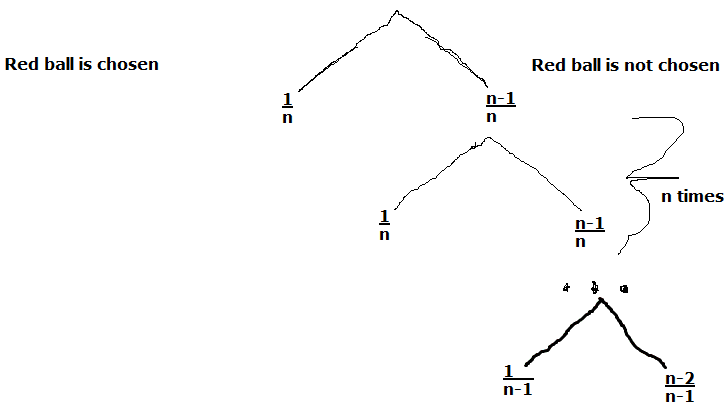# Probability Urn Problem with Replacement Kinda

• RaeganW

#### RaeganW

This probabilily summer course I am in is so bizarre; I have no idea where the professor is going during the lecture. Luckily I have awesome notes from another Prob & Stats course, but this problem has me stumped.

## Homework Statement

Consider two urns. The fi rst one has N red balls and the second one has N blue balls. Balls are removed randomly from urn 1 in the following manner: after each removal from urn 1, a ball is taken from urn 2 (if urn 2 still has balls) and placed in urn 1. The process continues until all balls have been removed. What is the probability that the last ball removed from urn 1 is red?

Hint: consider first one particular red ball and compute the probability that it is the last one to be removed.

## Homework Equations

P(Bi|A) = [P(A|Bi)*P(Bi)] / Ʃ 1->n P(A|Bi)*P(Bi)

Maybe?

## The Attempt at a Solution

I figured out there will be 2n trials. During the first n trials there are n balls in urn 1, in the n+1th trial there are n-1 balls in urn 1, n+2th trial there are n-2 balls in urn 1, etc so the 2nth trial will draw the last ball out of urn 1.

In my other course, we didn't really get into Bayesian probability. We were shown how to make a tree, but we've never gone over that in this class so I don't know if the professor will accept that kind of answer. But, for a specific red ball to be drawn last:Hope that makes sense... branches to the left are the probability the specific red ball is chosen, branches to the right is the probability the specific red ball is not chosen.

For the first n trials, the probability that the specific red ball is not chosen is ((n-1)/n)^n.
For the second n trials, the probability that the specific red ball is not chosen is ∏ 1-> (n-1) 1-(1/(n-i)) which I know isn't in the image but I just figured out that's a better way of writing it.
The 2nth trial the probability of choosing the specific red ball is 1, it's the last ball.

But that's only for a particular red ball, not any red ball. No idea how to scale this up to that, and I'm pretty sure there's a more succinct way of doing this... one that doesn't involve drawing so many pictures...

I think you are close to the answer, and I don't think you need Baye's Rule.

You have already figured out that the probability the designated red ball is not chosen in the first n draws is $\left( \frac{n-1}{n} \right) ^n$.

So assume the ball has not been drawn yet, after n draws. There are still n balls in urn 1.
What is the probability it is not drawn on the n+1 th draw?

Now there are n-1 balls left in urn 1.
What is the probability the special red ball is not drawn on the n+2 th draw?